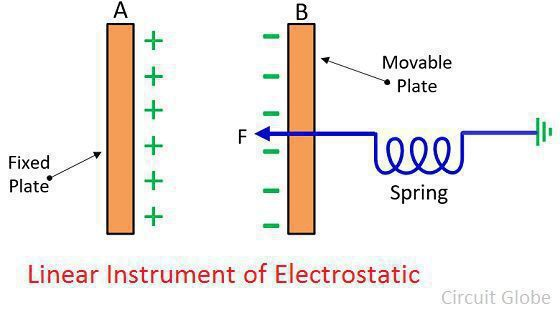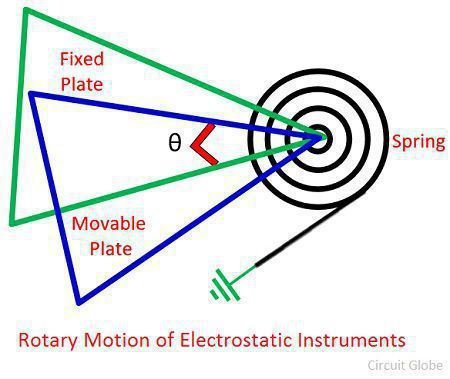# Electrostatic Instrument

Definition: The instrument whose working depends on the principle of attraction or repulsion of electrodes that carry electrical charges such type of instrument is known as the electrostatic instrument. In other words, the instrument which uses the static electric field for producing the deflecting torque is known as the electrostatic instrument. The electrostatic instrument is used for measuring the high and low voltage and also the power of the given circuit.

The electrostatic instrument works on the principle of mechanical interaction of the electrodes that consists the opposite electrical charge. The quantity which is measured by the electrostatic instrument is converted into either AC or DC voltage.

There are two ways of constructions of electrostatic instruments;

1. In the electrostatic instrument, the charge is stored between the plates. The electrostatic instrument consists the charges of two opposite polarity and force of attraction occurs between these two plates. Because of the force of attraction, the movable plates move towards the fixed plates and store the maximum electrostatic energy.
2. In this type of instruments, there are forces of attraction or repulsion occur between the rotary plate.

## Force and Torque Equations of Electrostatic Instruments

### Linear Type Electrostatic Instrument

The figure below shows the linear electrostatic type instrument. The plates A become positively charged, and the plate B becomes negatively charged. The positive charge plates become fixed, and the negative plates become movable. The spring is connected to the negatively charged plates for controlling the movement.When the voltage is applied to the plate, then the force of attraction induces between them. The plate tries to moves towards A until the force becomes maximum. The C is the capacitance (in farad) between the plate. The expression gives the total energy stores between the plates.

### Rotary type Electrostatic Instrument

This type of instrument carries the rotary plates. Because of the movement of the rotary plate, the force of attraction or repulsion occurs between them.1. Both the AC and DC voltage can be measured by using the electrostatic instrument.
2. The electrostatic type instrument consumes very less power.
3. The high value of voltage can be measured by using the instrument.
4. In the rotary type electrostatic instrument, in spite of linear displacement, the angular displacement occurs between the fixed and the moving plates.
5. The instrument has less Waveform and frequency error.
6. No error occurs because of the stray magnetic field.
7. The instrument is designed for large voltage.

### Disadvantages of Electrostatic type instrument

1. The non-uniform scale is used in the instrument.
2. The force of very small magnitude involves in the instrument.
3. The instrument is quite costly as compared to the other instrument.
4. The size of the instrument is also very large.

The reading of the electrostatic instrument is free from frequency.

### 5 thoughts on “Electrostatic Instrument”

1. manojbendarkar@gmail.com

I am Manish B. And it’s has been a month that is am searching for it.thanks for providing such a nice info .